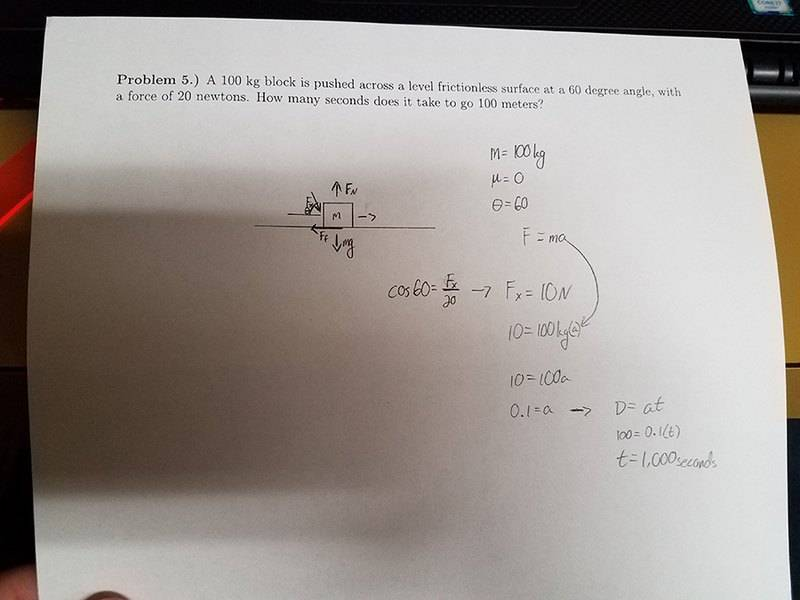# How long does it take to push this block at an angle?

## Homework Statement

A 100 kg block is pushed across a level frictionless surface at a 60 degree angle, with a force of 20 newtons. How many seconds does it take to go 100 meters?

m = 100kg
Mu = 0

F = MA

## The Attempt at a Solution

I sketched out the problem on my paper here, showing the downward angle force of 20N as Fx, and then the angle of 60 degrees being theta. From there it just turned into a mess of equations, some of which may be right, others which may not, and I was wondering if I did this problem correctly.Student100
Gold Member

## Homework Statement

A 100 kg block is pushed across a level frictionless surface at a 60 degree angle, with a force of 20 newtons. How many seconds does it take to go 100 meters?

m = 100kg
Mu = 0

F = MA

## The Attempt at a Solution

I sketched out the problem on my paper here, showing the downward angle force of 20N as Fx, and then the angle of 60 degrees being theta. From there it just turned into a mess of equations, some of which may be right, others which may not, and I was wondering if I did this problem correctly.You have the wrong equation for the last part. What is kinematic equation for position?

gneill
Mentor
The problem is vague in that it doesn't specify how the angle is to be measured. Is it measured from the vertical? From the horizontal? It makes a difference.

haruspex
Homework Helper
Gold Member
2020 Award
The problem is vague in that it doesn't specify how the angle is to be measured. Is it measured from the vertical? From the horizontal? It makes a difference.
.. and is its vertical component up or down?

The problem is vague in that it doesn't specify how the angle is to be measured. Is it measured from the vertical? From the horizontal? It makes a difference.
Welcome to my world with my physics professor that drives me insane. I believe it's supposed to be how it is in the attached drawing. 60 degrees upward from the x axis.

haruspex
Homework Helper
Gold Member
2020 Award
30 degrees upward from the x axis
The drawing has it acting downward from 30 degrees above the x axis. I assume that is what you mean.

You have the wrong equation for the last part. What is kinematic equation for position?

Ohh, it's D = InitialVelocity*Time + 0.5a*t^2, isn't it?

haruspex